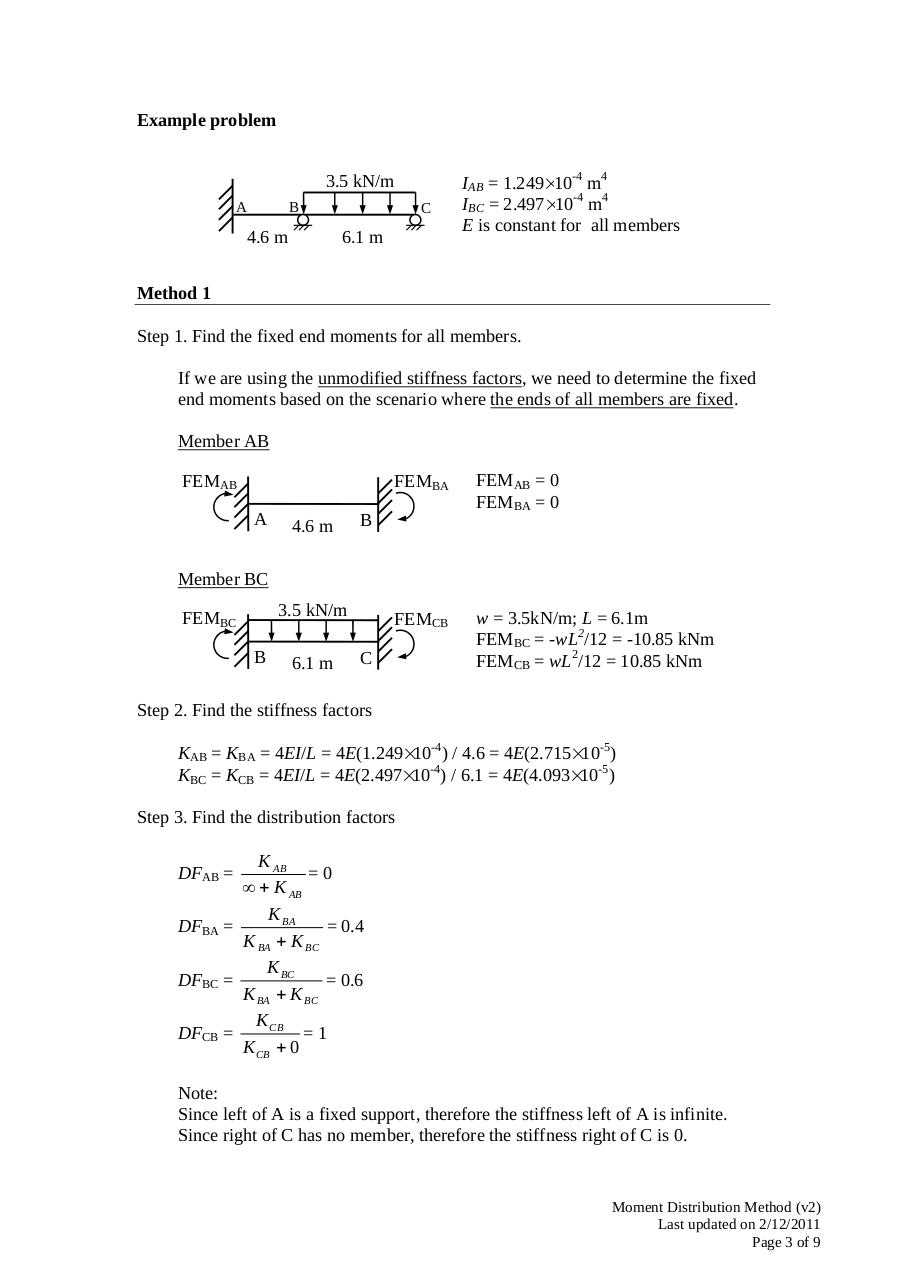# TDII MDM (v2).pdfPage 1 2 3 4 5 6 7 8 9

#### Text preview

Example problem
3.5 kN/m
A

B

4.6 m

C

6.1 m

IAB = 1.249×10-4 m4
IBC = 2.497×10-4 m4
E is constant for all members

Method 1
Step 1. Find the fixed end moments for all members.
If we are using the unmodified stiffness factors, we need to determine the fixed
end moments based on the scenario where the ends of all members are fixed.
Member AB
FEMAB
A

4.6 m

FEMBA

FEMAB = 0
FEMBA = 0

FEMCB

w = 3.5kN/m; L = 6.1m
FEMBC = -wL2/12 = -10.85 kNm
FEMCB = wL2/12 = 10.85 kNm

B

Member BC
3.5 kN/m

FEMBC
B

6.1 m

C

Step 2. Find the stiffness factors
KAB = KBA = 4EI/L = 4E(1.249×10-4) / 4.6 = 4E(2.715×10-5)
KBC = KCB = 4EI/L = 4E(2.497×10-4) / 6.1 = 4E(4.093×10-5)
Step 3. Find the distribution factors

K AB
=0
∞ + K AB
K BA
= 0.4
DFBA =
K BA + K BC
K BC
DFBC =
= 0.6
K BA + K BC
K CB
DFCB =
=1
K CB + 0
DFAB =

Note:
Since left of A is a fixed support, therefore the stiffness left of A is infinite.
Since right of C has no member, therefore the stiffness right of C is 0.

Moment Distribution Method (v2)
Last updated on 2/12/2011
Page 3 of 9